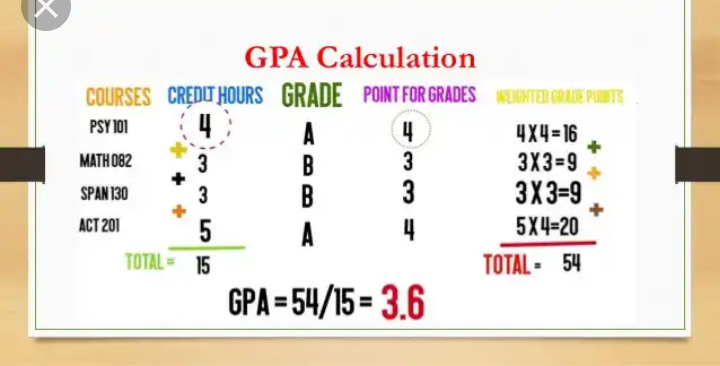Sign inEducation

College Education

# How to calculate GPA as a student in your various institutes.

##The formula for calculating GPA is as following.

Sum of (weight Grade point x credit unit) / sum of ( credit unit).

Alternatively, the formula could be expressed as:

Total aggregate grade point per semester/ total number of credit unit per semester

Where the total aggregate grade point per semester is obtained by multiplying the weighted point for each course offered in a semester is obtained by summing up all the course offered in a semester.

## Worked example:

Ade offer six-course in this semester.so let solve is it

BFN 111. ----76. ----A. -----4.00. ----2. ---6.00

BFN 112. ---62. --Bc. ---3.00.---- 3. ---9.00

BFN 113. ----43.---- E. ----2.00.--- 2. 4.00

BFN 114. ----78.---- A. ----4.00. -----3. ---12.00

GNS111.---- 65.---- B.----- 3.25. ---2. ---6.50

GNS113. --54. ---CD.--- 2.50.--- 2. ---5.00

Total.= 14. -----42.50

Given that formula for calculating GPA, Ade's GPA for the first semester is: and

Total aggregate Grade point per semester /total number of credit unit. = 42.50/14. = 3.35 or 3.04

The GPA of Ade Second semester.

BFN121. ---65. -----B.---- 3.25.---- 3. ----9.75

BFN122. ---42.--- E. ----2.00. ----2. 4.00

BFN 131. ----51. ---CD. ----2.50. ---3.--- 7.50

BFN132.---- 57. ----C.---- 2.75. -----3.---- 8.25

GNS121. ----71. ----AB.---- 3.50. ---3.--- 10.5

GNS122. -----62.---- Bc.---- 3.00.---- 2. ----6.00

Total.= 16. -----46

The GPA for Ade second semester is :

= 42 / 16. = 2.88.

## Cumulative Grade point Average. (CGPA)

The CGPA is calculated as the summation of a student's GPA for two or more.

If we consider the GPA for Ade in the first and second semester, 2018/2019.

CGPA for Ade could be calculated like this:

3.04. +. 2.88 / 2. = 5.92/2. = 2.96

## Final CGPA. ----- class of degree

3.5 - 4.00 ------------------Distinction

3.00 - 3.49------------------- Upper Credit

2.50 - 2.99------------ Lower credit

2.00 - 3.49----------------- Pass

Below - 2.00 ----------------Fail

Please keep following me up and I will update you with the best. Don't forget to like, comment and share is just a second.

Content created and supplied by: Defreelord (via Opera News )